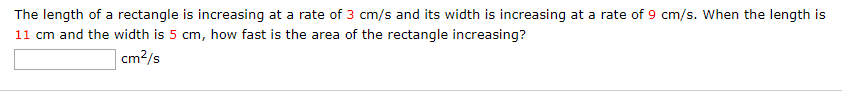# The length of a rectangle is increasing at a rate of 3 cm/s and its width is increasing at a rate of 9 cm/s. When the length is11 cm and the width is 5 cm, how fast is the area of the rectangle increasing?cm?/s

Question
2 viewshelp_outlineImage TranscriptioncloseThe length of a rectangle is increasing at a rate of 3 cm/s and its width is increasing at a rate of 9 cm/s. When the length is 11 cm and the width is 5 cm, how fast is the area of the rectangle increasing? cm?/s fullscreen
check_circle

Step 1

Consider the given situation,

Let the length of the rectangle be ‘l’ and breadth be ‘b’.

Area of rectangle is given as product of length and breadth.

Step 2

To calculate the rate of change of area, we will have to make an equation using the equation ...

### Want to see the full answer?

See Solution

#### Want to see this answer and more?

Solutions are written by subject experts who are available 24/7. Questions are typically answered within 1 hour.*

See Solution
*Response times may vary by subject and question.
Tagged in

### Derivative